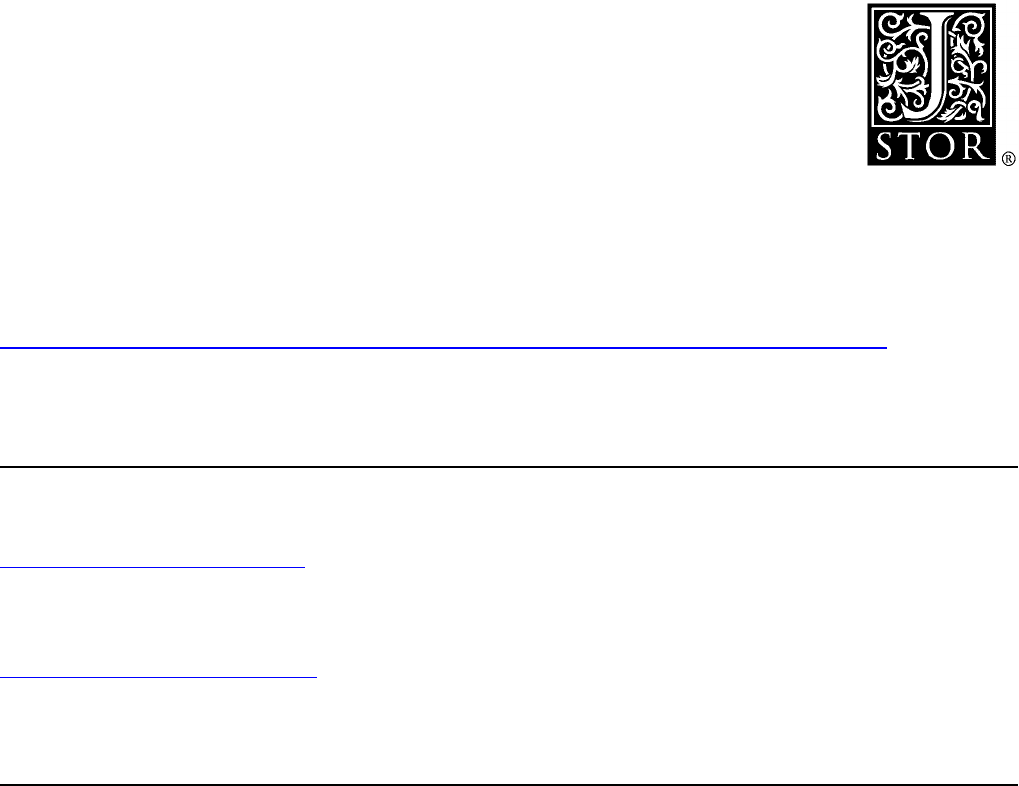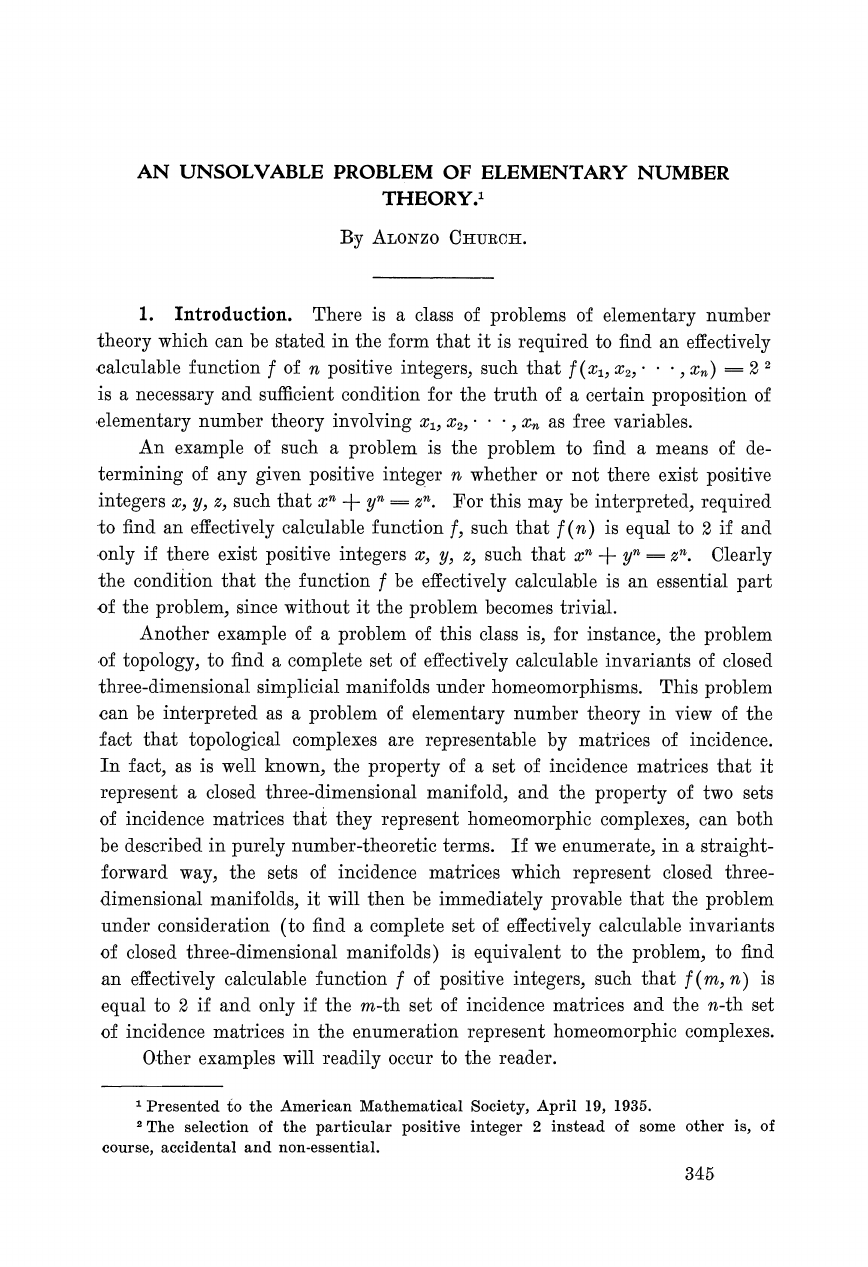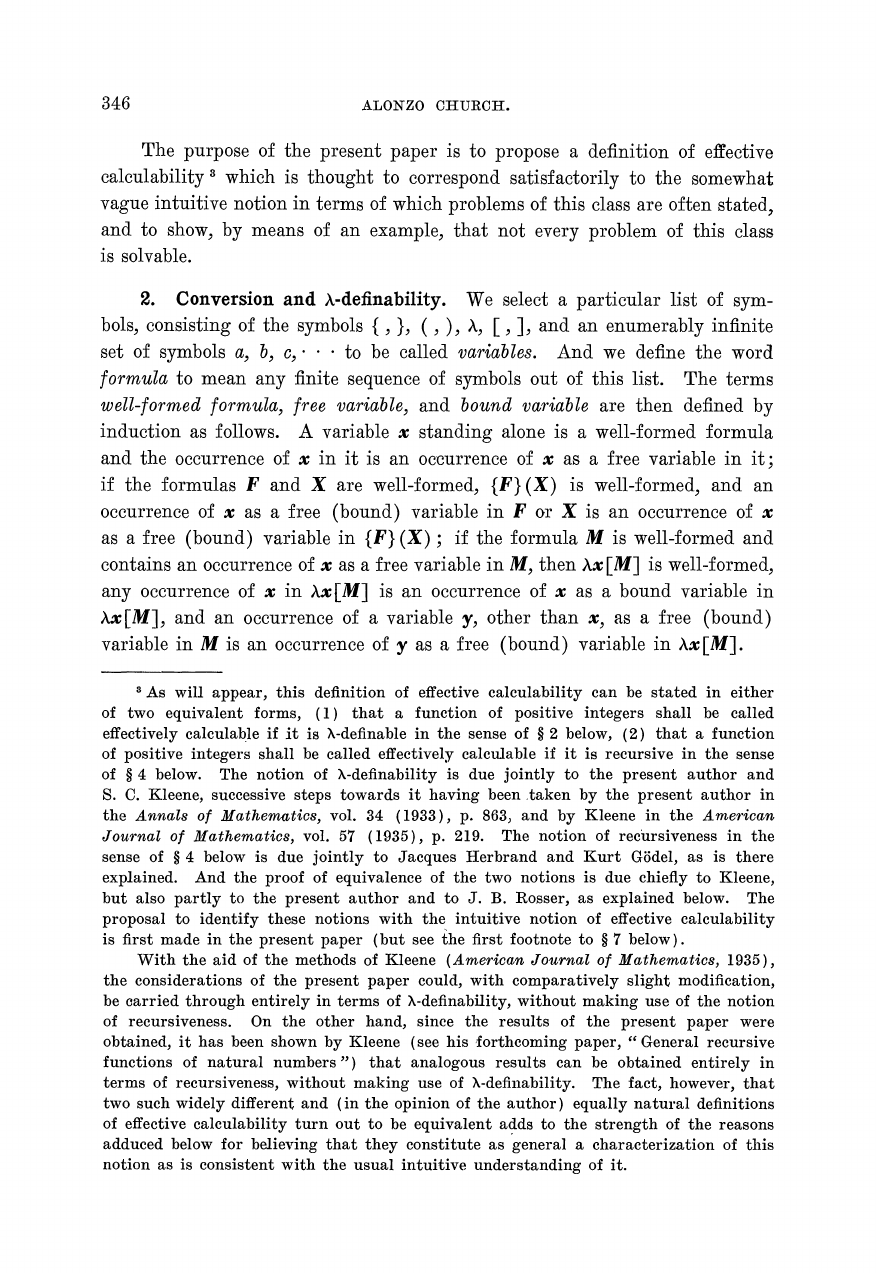An Unsolvable Problem of Elementary Number Theory
Alonzo Church
American Journal of Mathematics, Vol. 58, No. 2. (Apr., 1936), pp. 345-363.
Stable URL:
American Journal of Mathematics is currently published by The Johns Hopkins University Press.
Your use of the JSTOR archive indicates your acceptance of JSTOR's Terms and Conditions of Use, available at
http://www.jstor.org/about/terms.html. JSTOR's Terms and Conditions of Use provides, in part, that unless you have obtained
prior permission, you may not download an entire issue of a journal or multiple copies of articles, and you may use content in
the JSTOR archive only for your personal, non-commercial use.
Please contact the publisher regarding any further use of this work. Publisher contact information may be obtained at
http://www.jstor.org/journals/jhup.html.
Each copy of any part of a JSTOR transmission must contain the same copyright notice that appears on the screen or printed
page of such transmission.
journals and scholarly literature from around the world. The Archive is supported by libraries, scholarly societies, publishers,
and foundations. It is an initiative of JSTOR, a not-for-profit organization with a mission to help the scholarly community take
http://www.jstor.org
Mon Mar 3 10:49:53 2008AN UNSOLVABLE PROBLEM OF ELEMENTARY NUMBER
THEORY.=
1.
Introduction.
There is a class of problems of elementary number
theory which can be stated in the form that it is required to find an effectively
calculable function
f
of n positive integers, such that
f
(x,, x,,
.
. .
,
x,)
=
2
is a necessary and sufficient condition for the truth of a certain proposition of
elementary number theory involving x,, x,,
. .
.
,
x, as free variables.
An example of such a problem is the problem to find a means of de-
termining of any given positive integer n whether or not there exist positive
integers
2,
y,
z,
such that xn
-/-
yn
=
xn.
For this may be interpreted, required
to find an effectively calculable function
f,
such that
f
(n) is equal to
2
if and
only if there exist positive integers x, y,
z,
such that xn
+
yn
=
xn.
Clearly
the condition that the function
f
be effectively calculable is an essential part
of the problem, since without it the problem becomes trivial.
Another example of a problem of this class is, for instance, the problem
of topology, to find a complete set of effectively calculable invariants of closed
three-dimensional simplicia1 manifolds under homeomorphisms. This problem
can be interpreted as a problem of elementary number theory in view of the
fact that topological complexes are representable by matrices of incidence.
In fact, as is well known, the property of a set of incidence matrices that it
represent a closed three-dimensional manifold, and the property of two sets
of incidence matrices that they represent homeomorphic complexes, can both
be described in purely number-theoretic terms. If we enumerate, in a straight-
forward way, the sets of incidence matrices which represent closed three-
dimensional manifolds,
it
will then be immediately provable that the problem
under consideration (to find a complete set of effectively calculable invariants
of closed three-dimensional manifolds) is equivalent to the problem, to find
an effectively calculable function
f
of positive integers, such that
f
(m, lz) is
equal to
2
if and only if the m-th set of incidence matrices and the lz-th set
of incidence matrices in the enumeration represent homeomorphic complexes.
Presented to the American Mathematical Society, April
19,
1935.
The selection of the particular positive integer
2
instead of some other is, of
course, accidental and non-essential.
345346
ALONZO
CHURCH.
The purpose of the present paper is to propose a definition of effective
calculability
which is thought to correspond satisfactorily to the somewhat
vague intuitive notion in terms of which problems of this class are often stated,
and to show, by means of an example, that not every problem of this class
is solvable.
2.
Conversion and A-definability. We select a particular list of sym-
bols, consisting of the symbols
{
,
),
(
,
),
X,
[
,
1,
and an enumerably infinite
set of symbols a,
b,
c,
.
.
.
to be called variables. And we define the word
formula to mean any finite sequence of symbols out of this list. The terms
well-formed formula, free variable, and bound variable are then defined by
induction as follows.
A
variable x standing alone is a well-formed formula
and the occurrence of x in it is an occurrence of
x
as a free variable in it;
if the formulas
F
and X are well-formed, {F)(X) is well-formed, and an
occurrence of x as a free (bound) variable in
F
or
X
is an occurrence of
x
as a free (bound) variable in {F) (X)
;
if the formula M is well-formed and
contains an occurrence of
x
as a free variable in M, then hx[M] is well-formed,
any occurrence of x in h[M] is an occurrence of x as a bound variable in
hx[M], and an occurrence of a variable
y,
other than x, as a free (bound)
variable in M is an occurrence of
y
as a free (bound) variable in hx[M].
As will appear, this definition of effective calculability can be stated in either
of two equivalent forms, (1) that a function of positive integers shall be called
effectively calculable if
it
is h-definable in the sense of $2 below, (2) that a function of positive integers shall be called effectively calculable if it is recursive in the sense of$
4
below. The notion of h-definability is due jointly to the present author and
S.
C.
Kleene, successive steps towards it having been taken by the present author in
the Annals of Mathematics, vol.
34 (1933), p. 863, and by Kleene in the American
Journal
of
Mathematics, vol. 57 (1935), p. 219.
The notion of recursiveness in the
sense of
$4 below is due jointly to Jacques Herbrand and Kurt Gijdel, as is there explained. And the proof of equivalence of the two notions is due chiefly to Kleene, but also partly to the present author and to J. B. Rosser, as explained below. The proposal to identify these notions with the intuitive notion of effective calculability is first made in the present paper (but see the first footnote to$7 below).
With the aid of the methods of Kleene (American Journal of Mathematics, 1935),
the considerations of the present paper could, with comparatively slight modification,
be carried through entirely in terms of X-definability, without making use of the notion
of recursiveness. On the other hand, since the results of the present paper were
obtained, it has been shown by Kleene (see his forthcoming paper, "General recursive
functions of natural numbers") that analogous results can be obtained entirely in
terms of recursiveness, without making use of X-definability. The fact, however, that
two such widely different and (in the opinion of the author) equally natural definitions
of effective calculability turn out to be equivalent adds to the strength of the reasons
adduced below for believing that they constitute as general a characterization of this
notion as is consistent with the usual intuitive understanding of it.